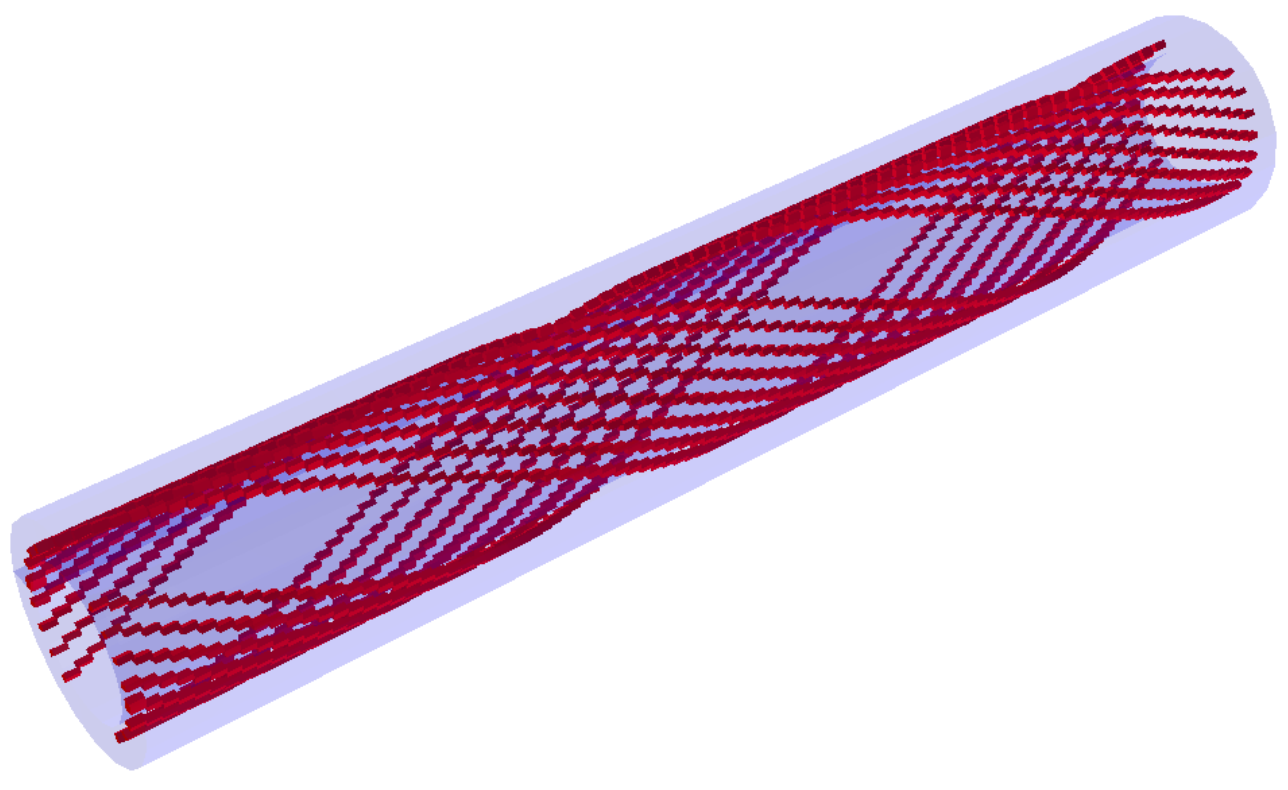# Beamline magnet elements¶

These detector elements don’t directly serve a role in particle detection, but have to be included to accomodate for particle-matter interactions. The provided magnet types are dipole and quadrupole magnets and spin rotators.

## Dipole magnets¶

Magnets generating dipole field used for beam steering and spectrometry.

example use:

``` <detector id="3" name="B0PF_BeamlineMagnet" type="B0pFMag">
<placement x="B0PF_XPosition" y="0*m" z="B0PF_CenterPosition" theta="B0PF_RotationAngle" />
<coil dx="0.1*m" dy="0.03*m" />
</detector>
```

Magnets used for bea focusing, loosely based on design shown here. More information can be also found at reference discussing the EIC design.

example use:

```<detector id="1" name="quad_example" vis="RedVis" type="QuadMagnet">
<placement x="0*m" y="0*m" z="0*m" theta="0.0" />
<dimensions r="0.2*m" z="3.8*m" />
<coil dx="8*cm" dy="2.5*cm" />
<apperture r="0.131*m" />
</detector>
```

## Helical dipole magnets¶

These beamline elements are used for spin rotation. See this reference for more information.example use:

```<detector id="1" name="spinp_example" vis="RedVis" type="HelicalDipole">
<placement x="0*m" y="0*m" z="0*m" theta="0.0" psi="0.0" />
<dimensions r="0.2*m" z="2.3*m" />
<coil dy="2.5*cm" />
<apperture r="0.131*m" />
</detector>
```